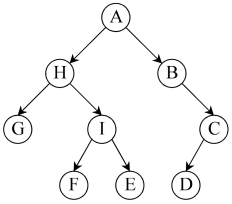# What is the Difference Between Tree and Binary Tree

The main difference between tree and binary tree is that tree arranges data in a structure similar to a tree, in a hierarchical manner, while a binary tree is a type of tree in which a parent node can have a maximum of two child nodes.

A data structure is a way of organizing data in a systematic way. This arrangement helps to increase the efficiency of programs. One common nonlinear data structure is called Tree. A binary tree is a type of tree.

### Key Areas Covered

1. What is a Tree
– Definition, Functionality
2. What is a Binary Tree
– Definition, Functionality
3. What is the Relationship Between Tree and Binary Tree
– Outline of the Association
4. What is the Difference Between Tree and Binary Tree
– Comparison of Key Differences

### Key Terms

Binary Tree, Nonlinear Data Structure, Tree## What is a Tree

A tree is a data structure that organizes the data in a tree-like structure. The data elements are called nodes of the tree. The main node is the root node, and all other elements (child nodes) are arranged under that node.  The section left to the root node and left to the right nodes are separate subtrees.Edges help to link the nodes in the tree. When a node connects to a specific node from the upward direction, that upward node is called the parent node. When a node connects to a specific node from the downward direction, that downward node is a child node. Moreover, a tree maintains a parent-child relationship among the node. A parent node can have multiple child nodes, but a child node can only have one parent node. However, some nodes do not connect to child nodes. We call these nodes leaf nodes.

## What is a Binary Tree

A binary tree is one type of tree. In these trees, each node can have a maximum of two child nodes. It also has the same properties as a usual tree. The topmost node is the root node. The nodes connect together according to the parent-child relationship. A node that has no child nodes is a leaf node.The main traversal operations of a binary tree are as follows.

Pre-order traversal – Traverse the root node first and then the left subtree and right subtree. This process applies to each subtree recursively.

In order traversal – Traverse the left subtree first, then the root node and right subtree. This process applies to each subtree recursively.

Post-order traversal – Traverse the left subtree, then the right and the root node. This process applies to each subtree recursively.

## Relationship Between Tree and Binary Tree

• A binary tree is a type of tree.

## Difference Between Tree and Binary Tree

### Definition

A tree is a data structure that simulates a hierarchical tree structure, with a root value and subtrees of children with a parent node while a binary tree is a type of data structure where each parent node can have at most two child nodes. These definitions thus explain the fundamental difference between tree and binary tree.

### Number of Child Nodes

In a tree, a parent node can have multiple child nodes. However, in a binary tree, a parent node can have a maximum of two child nodes. Hence, this is the main difference between tree and binary tree.

### Conclusion

A tree is a data structure that has multiple nodes; one node is the root while the remaining nodes are the child nodes of the root. The binary tree is a type of tree. The main difference between tree and binary tree is that tree arranges data in a structure similar to a tree in a hierarchical manner while a binary tree is a type of tree in which a parent node can have a maximum of two child nodes.

##### Reference:

1. “Tree – Javatpoint.” Www.javatpoint.com, Available here.
2. “Binary Tree – Javatpoint.” Www.javatpoint.com, Available here.

##### Image Courtesy:

1. “Trie example” By Booyabazooka (based on PNG image by Deco). Modifications by Superm401. – own work (based on PNG image by Deco) (Public Domain) via Commons Wikimedia
2. “Binary tree (letters)” (Public Domain) via Commons Wikimedia